## Recommended Posts

For this challenge, I've decided to go with the parabola yet again. If we take a triangle, it is possible to find three parabolic curves that fit perfectly inside the triangle such that two vertices of the triangle are located on a parabola with two sides of the triangle being tangent to the parabola at those points. The following image illustrates this property: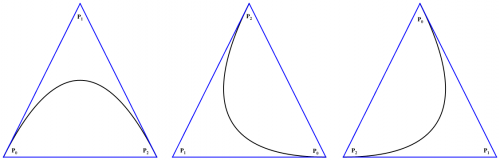The above image demonstrates these parabolic curves that fit perfectly in the triangle. Of course, the equation that describes the parabolas is not a standard function and is defined parametrically. Furthermore, the order of the points matter, which determines the parabolic curve and direction along the path. The goal of this challenge is to find the parametric equation that describes the parabolic curves that fit inside a triangle specified by the vertices $P_0=(x_0, y_0)$, $P_1=(x_1, y_1)$, and $P_2=(x_2, y_2)$.

Edited by Daedalus

##### Share on other sites

I appreciate the rep points, but is anyone interested in trying to solve the challenge? I do realize that it may seem complicated, but the answer can be derived using a few concepts from Calculus 1 and algebra.

Edited by Daedalus

##### Share on other sites

This seems like one I should be able to solve but I'm unsure if the antiderivative will give me the right function to parametrize.

I think you are heading in the wrong direction. Look further into what is going on in the problem. We clearly have two sides of the triangle that are tangent to the parabolic curve. To give you a hint, I had to use a limit. However, I didn't use the derivative directly. Instead, I used the tangents with a method I developed to solve this problem : ) I've never heard of anyone using this method before, but that doesn't mean it is new. Regardless, It's actually a pretty cool way to derive the parametric equation.

Edited by Daedalus

##### Share on other sites

Well, I'm stumped.I was hoping to use the slope of the tangent ( m ) with y = mx + Constant to get the derivative of the parabola at (x,y). Right now I'm trying to get Python to plot it in matplotlib
but mostly just reading the documentation for matplotlib.

I'm looking forward to seeing your solution.

##### Share on other sites

Well, I'm stumped.I was hoping to use the slope of the tangent ( m ) with y = mx + Constant to get the derivative of the parabola at (x,y). Right now I'm trying to get Python to plot it in matplotlib

but mostly just reading the documentation for matplotlib.

I'm looking forward to seeing your solution.

Now you are getting on the right track, and you already have two lines that are tangent to the parabolic curve. You should explore that method and see what you discover.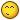Here, this should help you along:

Point-Slope Linear Form

$y-y_1=m(x-x_1)$

or

$y=m(x-x_1)+y_1$

Edited by Daedalus

##### Share on other sites

Can we assume that P0=-P2?

##### Share on other sites

Can we assume that P0=-P2?

It has to work for any triangle, but it can help to work with a simple triangle to solve the problem. To give you all another hint, I solved the problem much like one would do for approximating an integral or for arc length. Hence, the use of the limit.

Edited by Daedalus

##### Share on other sites

Once we know the derivative, can't we find the antiderivative at a point on the parabola to get the equation? I'm unsure about integrals.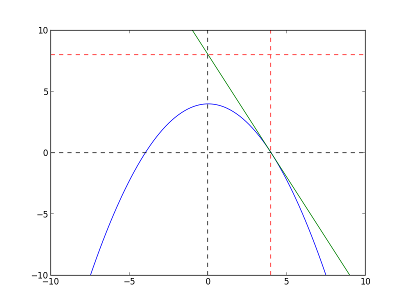##### Share on other sites

Once we know the derivative, can't we find the antiderivative at a point on the parabola to get the equation? I'm unsure about integrals.

I didn't use the derivative directly. Think about the other properties that tangent lines have besides being tangent to the curve. In other words, how can we use tangent lines to derive the parametric equation without using the derivative directly.

##### Share on other sites

Do we get a prize?

##### Share on other sites

Do we get a prize?

I will give you rep and 5 stars 1 additional star if you can solve the problem, and you might get rep and stars from other people too. However, the biggest prize is the knowledge and satisfaction that you gain from working the problem and finding the solution, which is why I post these challenges. Of course, it also allows me to share problems that I have solved in a fun way instead of just posting a bunch of equations.

Edit: Perhaps five stars is a little too much for solving one problem. I'll give you an extra star for each challenge that you win. By the time you have completed five challenges, you will have a vote of five stars and five points of rep from me. I realize it isn't much of a prize, but it's the best I can do. If I could offer more rep for solving certain challenges, I would. At least you will have gained some knowledge and bragging rights for being the first person to solve each challenge.Edited by Daedalus

##### Share on other sites

//I can get pretty close with linear approximation, but I don't know how to say it in Math.
#include "stdio.h"

//f(x) = f(a) + f'(a)(x - a)

int main(int argc, char *argv[])
{
float X = -2; //startpoint a=(-2,0)
float slope = -2; //f'(a)
float Y =0; //f(a)
float dx=0.01; //interval

printf("%f\n",Y);
for(X=-2;X<=2;X+=dx){
Y = Y + slope*X*dx;
printf("%f\n",Y);
}
return 0;
}

Thanks for another enlightening challenge!

##### Share on other sites

Thanks for another enlightening challenge!

You're welcome. At risk of giving the solution away, I'm going to give you one last hint. You have 2 vertices of the triangle that are also points on the parabola. You also have two sides of the triangle that are tangent to the parabola at those 2 vertices. Now, you have the third vertex of the triangle that has nothing to do with the parabolic curve. The only thing this third point has in common with the parabola is that it is also where both tangent lines intersect. I have now given you most of the information needed to solve the challenge. If you read the thread carefully, you should be able to use this hint to figure out how to find the solution.

If no one replies to the thread asking for more time by tomorrow, I will go ahead and post the solution.Edited by Daedalus

##### Share on other sites

Can we have more time?

##### Share on other sites

Odd as it may seem I was trying to solve a similar problem at work a while ago.

I was trying to predict the melting points of mixtures of 2 materials, given very little starting data.

What I had was the melting points of the 2 materials and their cryoscopic constants.

Here is the world's worst diagram

Anyway, I had the gradients at the two extremes of the range and I had the values at those ends too.

I ended up fitting a cubic equation to the data because a quadratic wouldn't fit.

I was then able to estimate the melting points of the mixtures in between.

the point is that I couldn't get a quadratic to work- there was "too much" data.

So, I suspect that the answer to the question is no.

I can draw a parabola through a point with a given gradient at that point and which passes through a second point, but I have run out of variables to decide what the gradient will be at that second point.

I think you would need to use cubics, rather than parabolae.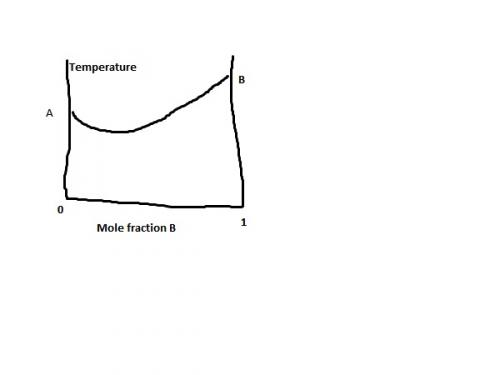##### Share on other sites

Can we have more time?

You sure can. I'll give you till Dec 2, 2013.

Odd as it may seem I was trying to solve a similar problem at work a while ago.

I was trying to predict the melting points of mixtures of 2 materials, given very little starting data.

What I had was the melting points of the 2 materials and their cryoscopic constants.

Here is the world's worst diagram

Anyway, I had the gradients at the two extremes of the range and I had the values at those ends too.

I ended up fitting a cubic equation to the data because a quadratic wouldn't fit.

I was then able to estimate the melting points of the mixtures in between.

the point is that I couldn't get a quadratic to work- there was "too much" data.

So, I suspect that the answer to the question is no.

I can draw a parabola through a point with a given gradient at that point and which passes through a second point, but I have run out of variables to decide what the gradient will be at that second point.

I think you would need to use cubics, rather than parabolae.

This particular problem actually results in a parabola. There is a very interesting property that tangent lines to parabolas have that make it possible to solve this one.

Edited by Daedalus

##### Share on other sites

Is it -| x * 31/2 * | x |1/2 * ( 1 / x2)1/2 | / 2 + 1.251/2/2, where |x| represents the absolute value, and assuming x0 = - x2?

##### Share on other sites

Is it -| x * 31/2 * | x |1/2 * ( 1 / x2)1/2 | / 2 + 1.251/2/2, where |x| represents the absolute value, and assuming x0 = - x2?

No, the actual solution is in parametric form where you have an equation for $x$ and one for $y$. An example would be:

$F_x(t)=t$

$F_y(t)=t^2$

Remember, the equation uses all three vertices of the triangle to define the parabolic curves, and the order of the points determine the curve (there are three) and direction along the path.

##### Share on other sites

Perhaps I need someone to shine a searchlight on this problem for me.

##### Share on other sites

After speaking with Endercreeper01, he's decided to go ahead and let me post the solution lol...Deriving the Solution

In order to solve this challenge, we'll need to partition two sides of the triangle into $n$ segments. We also have to account for direction. So, if we consider that an object moves along the curve from the point $P_0$ towards $P_1$ ending at $P_2$, then the first side, U, starts at $(x_0,y_0)$ and ends at $(x_1,y_1)$ and the other side, V, at $(x_1,y_1)$ to $(x_2,y_2)$. This allows us to define the following parametric equations that describe the points along the specified sides of the triangle in terms of our parameter $t$ where $\{t \in \mathbb{N} : 0\le t\le n\}$.

$\text{U}_x(t)=x_0+(x_1-x_0)\,\frac{t}{n}$

$\text{U}_y(t)=y_0+(y_1-y_0)\,\frac{t}{n}$

$\text{V}_x(t)=x_1+(x_2-x_1)\,\frac{t}{n}$

$\text{V}_y(t)=y_1+(y_2-y_1)\,\frac{t}{n}$

Now that we have the equations for the points along both sides of the triangle, we will use them to create an equation that describe the lines tangent to the parabola.

$\text{L}(t,x)=\frac{\text{V}_y(t)-\text{U}_y(t)}{\text{V}_x(t)-\text{U}_x(t)}\left(x-\text{U}_x(t)\right)+\text{U}_y(t)$

The following image illustrates these lines tracing out a parabolic curve.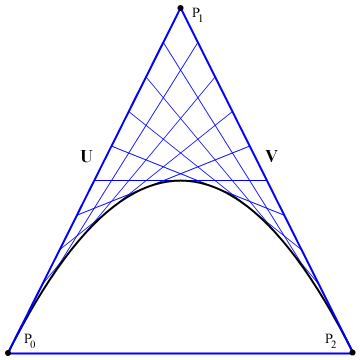We can see that the parabolic curve is approximated by the points at the intersection of two consecutive lines. As the number of partitions approach infinity, the points of intersection converge to the points on the parabolic curve. By solving $\text{L}(t)=\text{L}(t+1)$, we can derive the $x$ and $y$ equations that define the points of intersection. Working the math we get:

$x(t,n)=\frac{n^2\,x_0-n(2t+1)(x_0-x_1)+t(t+1)(x_0-2 x_1+x_2)}{n^2}$

$y(t,n)=\frac{n^2\,y_0-n(2t+1)(y_0-y_1)+t(t+1)(y_0-2 y_1+y_2)}{n^2}$

Now that we have the parametric equation that defines the points of intersection, we can take the limit of this equation as the number of partitions $n$ approach infinity and arrive at the parametric equation that describes the parabolic curve. However, before we can do this, we'll need to redfine the parameter $t$ such that it approaches $n$ on the interval [0,1]. By redefining the parameter as $n\times t$, when $t=0$ we get back $0$ and when $t=1$ we get back $n$. This allows us to parametetize the function using $n\times t$ where $\{t \in \mathbb{R} : 0\le t\le 1\}$ while covering the entire domain previously defined for $t$: $\{t \in \mathbb{N} : 0\le t\le n\}$.

$\lim_{n \to \infty}x(n\,t,n)=x_0-2\,x_0\,t+x_0\,t^2+2\,x_1\,t-2\,x_1\,t^2+x_2\,t^2$

$\lim_{n \to \infty}y(n\,t,n)=y_0-2\,y_0\,t+y_0\,t^2+2\,y_1\,t-2\,y_1\,t^2+y_2\,t^2$

After we combine like terms and simplify the result, we finally arrive at the solution to the challenge.

$P_x(t)=(x_0-2\,x_1+x_2)\,t^2-2(x_0-x_1)\,t+x_0$

$P_y(t)=(y_0-2\,y_1+y_2)\,t^2-2(y_0-y_1)\,t+y_0$

where $\{t \in \mathbb{R} : 0\le t\le 1\}$

Verifying the Result

We'll verify the solution by checking a known parabolic curve, $y=5-(1/5) x^2$. We'll choose the points (-5,0) and (5,0) on the parabola to derive the third point of the triangle. The slope of the parabola at $x$ is $m=-(2/5)x$. This allows us to use the point-slope form to find the lines that are tangent to the parabola at $x=-5$ and $x=5$. Using these values we get:

$T_0(x)=2(x+5)$

$T_1(x)=-2(x-5)$

Solving for the point of intersection we get:

$2(x+5)=-2(x-5)$

$x=0$
$y=2(0+5)=10$

Thus, the point in the middle, $P_1$, is located at (0,10). By using these points (-5,0), (0,10), and (5,0), we can check to see if the solution will produce the same parabolic curve. The following is a graph of both, $y=5-(1/5) x^2$ and the parametric equation using the specified points.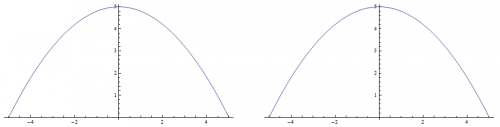By using a little algebra we can tranform the parametric equation back into a function and prove that both curves are equal. Basically, we use the parametric equation for the $x$ coordinate and solve for $t$ in terms of $x$. Then, we substitute that result into the parametric equation for the $y$ coodinate. The result is a standard function $y=f(x)$, which is equal to our test parabola. Thus, proving that these curves are in fact parabolas.

$x=((-5)-2\,(0)+(5))\,t^2-2((-5)-(0))\,t+(-5)=10\,t-5$

$t=\frac{x+5}{10}$

Substitute $t$ into the parametric equation for the $y$ coordinates.

$y=((0)-2\,(10)+(0))\,t^2-2((0)-(10))\,t+(0)=20\left(t-t^2\right)$

$y=20\left(\frac{x+5}{10}-\frac{(x+5)^2}{10^2}\right)=5-\frac{1}{5}x^2$

Interesting Shapes and Curves

If we reorder the vertices, then we can generate three different parabolic curves depending on the angles of the triangle and lengths of the sides.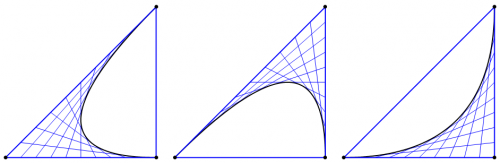If we take each curve, we can glue them together at their endpoints and generate a smooth closed region.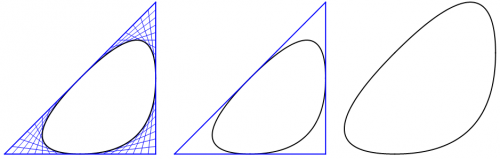We can also glue the end points together to form smooth piecewise curves.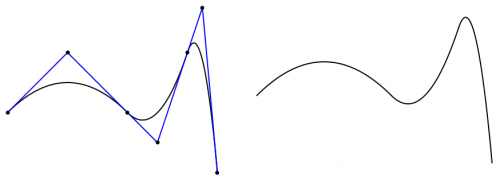However, I like this shape the best.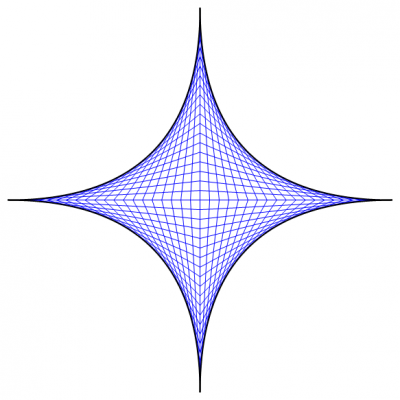As usual, I've attached the Mathematica 7 file for the challenge to this post.

TenthChallenge.zip

Edited by Daedalus

##### Share on other sites

I always seem to see the patterns in the numbers, and while playing around with the result of this challenge, I managed to extend the solution beyond parabolic curves:

$P_x(t)=(x_0-2\,x_1+x_2)\,t^2-2(x_0-x_1)\,t+x_0$

$P_y(t)=(y_0-2\,y_1+y_2)\,t^2-2(y_0-y_1)\,t+y_0$

where $\{t \in \mathbb{R} : 0\le t\le 1\}$

If you look at the above result, you should notice some patterns that seem to be a recurring theme. As a bonus, I'll give anyone rep who can tell me what type of curve this new pattern entails. It's interesting, because I should've noticed this when I first worked the problem##### Share on other sites

Is it a quadratic Bezier curve?

Mainly just Bézier curves in general. The solution to the original challenge is as you say, quadratic Bézier curves. The general equation can be found here at Wikipedia.

##### Share on other sites

I believe there can be many Parabolas and Ellipses which may fulfill the condition of being tangential at [X0, Y0] and [X1, Y1] in the given triangle with [X2, Y2] as its apex.

................. I have a power cut and will continue later

>>>>>>>>>>>>>>>

PS : After thinking over this problem for a while I wanted to give my views here and started writing the above.

Only then I saw the preceding posts and found that it appears to be settled / solved and taken care of my points too.

Therefore I have nothing more to give here just now

Edited by Commander

## Create an account

Register a new account